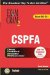Chapter 16. Answer Key 1

1. A, B, C

2. D

3. A, B

4. B

5. B

6. A

7. C, E

8. A

9. C

10. A, D

11. A, B, C, D, E

12. A, D

13. B

14. B, C, E

15. A, C, D

16. A, B, C, D

17. D

18. C

19. D

20. B, D

21. A

22. E

23. E

24. C

25. E

26. D

27. B

28. A, B, C

29. D

30. A

31. A

32. B

33. A, D

34. A, C

35. B

36. B

37. D

38. B, C

39. C

40. D

41. D

42. B

43. A

44. D

45. B, C

46. C

47. A

48. A, B, C, D

49. B

50. D

51. A, C

52. C

53. A, C, D

54. D

55. A, C, D